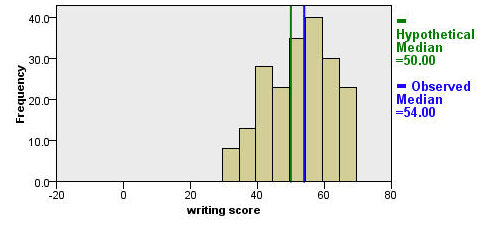## One sample median test

A one sample median test allows us to test whether a sample median differs significantly from a hypothesized value. We will use the same variable, write, as we did in the one sample t-test example above, but we do not need to assume that it is interval and normally distributed (we only need to assume that write is an ordinal variable).

nptests /onesample test (write) wilcoxon(testvalue = 50).